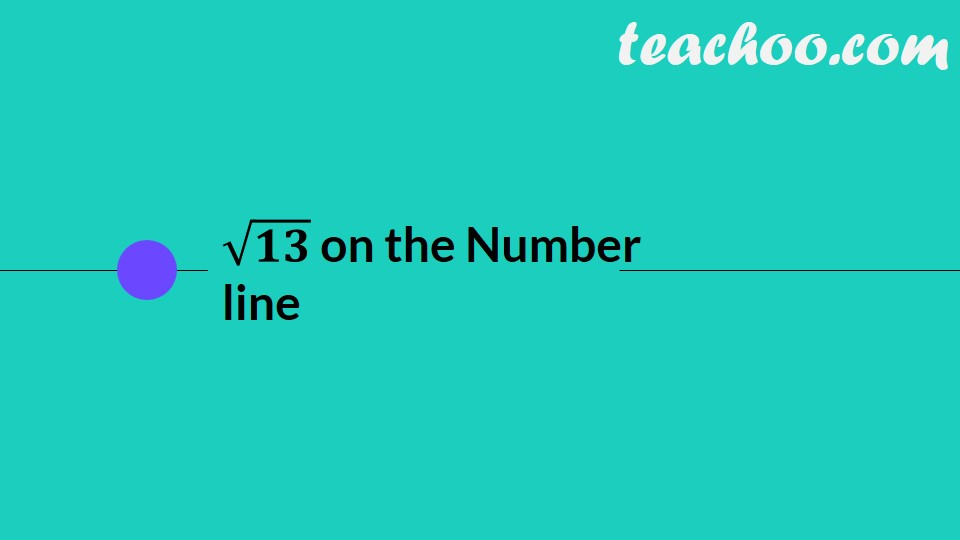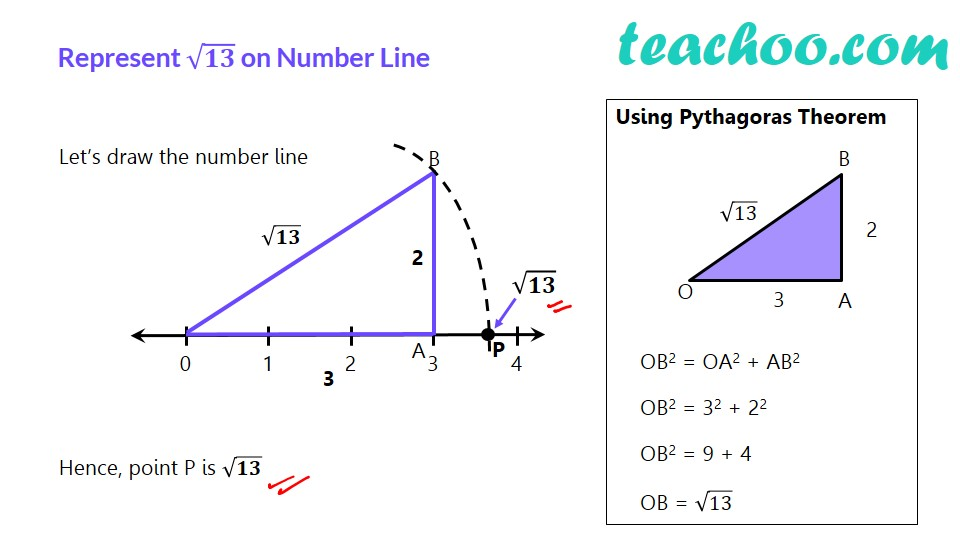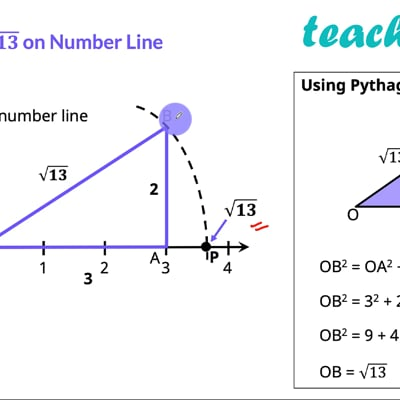Irrational Numbers

Chapter 1 Class 9 Number Systems
Concept wiseThis video is only available for Teachoo black users

Get live Maths 1-on-1 Classs - Class 6 to 12

### Transcript

√𝟏𝟑 on the Number line Represent √𝟏𝟑 on Number Line Let’s draw the number line Hence, point P is √𝟏𝟑 Using Pythagoras Theorem OB2 = OA2 + AB2 OB2 = 32 + 22 OB2 = 9 + 4 OB = √13